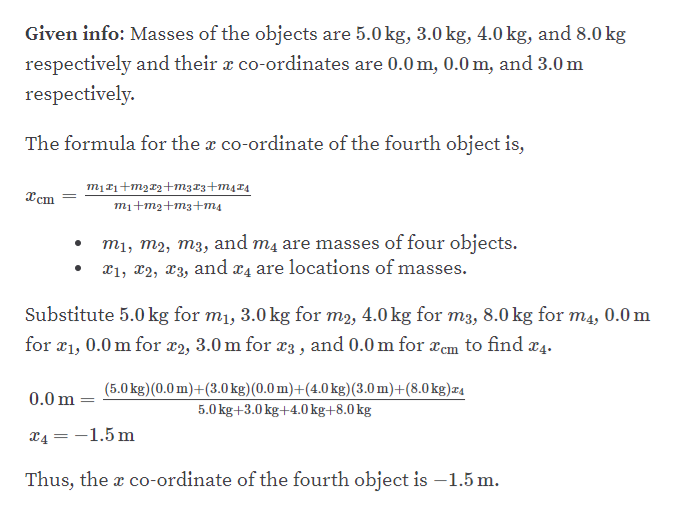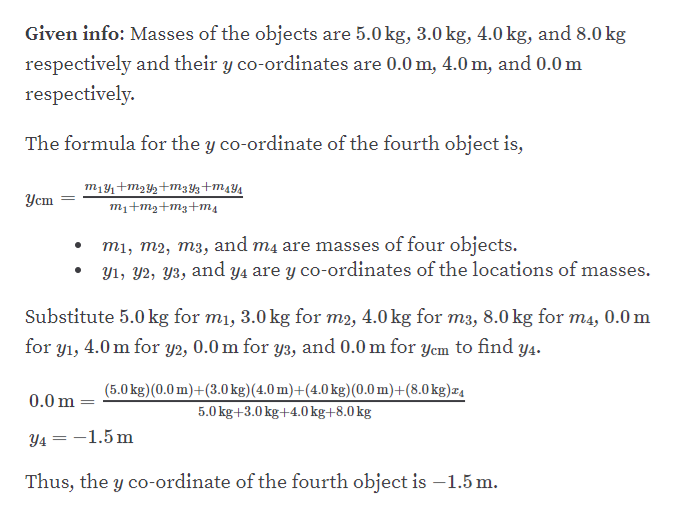# Consider the following mass distribution, where x- andy-coordinates are given in meters: 5.0 kg at (0.0, 0.0) m, 3.0 kgat (0.0, 4.0) m, and 4.0 kg at (3.0, 0.0) m. Where should afourth object of 8.0 kg be placed so that the center of mass ofthe four-object arrangement will be at (0.0, 0.0) m?

Question
363 views

Consider the following mass distribution, where x- and
y-coordinates are given in meters: 5.0 kg at (0.0, 0.0) m, 3.0 kg
at (0.0, 4.0) m, and 4.0 kg at (3.0, 0.0) m. Where should a
fourth object of 8.0 kg be placed so that the center of mass of
the four-object arrangement will be at (0.0, 0.0) m?

check_circle

Step 1help_outlineImage TranscriptioncloseGiven info: Masses of the objects are 5.0 kg, 3.0 kg, 4.0 kg, and 8.0 kg respectively and their x co-ordinates are 0.0 m, 0.0 m, and 3.0 m respectively. The formula for the x co-ordinate of the fourth object is, mị£1+m2¤2+mz13+m4T4 Xcm mi+mg+m3+т4 m1, m2, m3, and m4 are masses of four objects. x1, x2, x3, and x4 are locations of masses. Substitute 5.0 kg for m1, 3.0 kg for m2, 4.0 kg for m3, 8.0 kg for m4, 0.0 m for æ1, 0.0 m for r2, 3.0 m for x3 , and 0.0 m for xem to find x4. (5.0 kg)(0.0 m)+(3.0 kg)(0.0 m)+(4.0 kg)(3.0 m)+(8.0kg)z4 0.0 m 5.0 kg+3.0 kg+4.0 kg+8.0 kg X4 = -1.5 m Thus, the x co-ordinate of the fourth object is –1.5 m. fullscreen
Step 2help_outlineImage TranscriptioncloseGiven info: Masses of the objects are 5.0 kg, 3.0 kg, 4.0 kg, and 8.0 kg respectively and their y co-ordinates are 0.0 m, 4.0 m, and 0.0 m respectively. The formula for the y co-ordinate of the fourth object is, m14+m2Y½+m3 Y3+m4Y4 Уст m1+m2+m3+ms mi, m2, m3, and mą are masses of four objects. Y1, Y2, Y3, and y4 are y co-ordinates of the locations of masses. Substitute 5.0 kg for m1, 3.0 kg for m2, 4.0 kg for m3, 8.0 kg for m4, 0.0 m for yı, 4.0 m for y2, 0.0 m for y3, and 0.0 m for yem to find y4. (5.0 kg)(0.0 m)+(3.0 kg)(4.0 m)+(4.0 kg)(0.0 m)+(8.0kg)z4 0.0 m 5.0 kg+3.0 kg+4.0 kg+8.0 kg Y4 = -1.5 m Thus, the y co-ordinate of the fourth object is –1.5 m. fullscreen

### Want to see the full answer?

See Solution

#### Want to see this answer and more?

Solutions are written by subject experts who are available 24/7. Questions are typically answered within 1 hour.*

See Solution
*Response times may vary by subject and question.
Tagged in
SciencePhysics

### Centre of Mass and Centre of Gravity